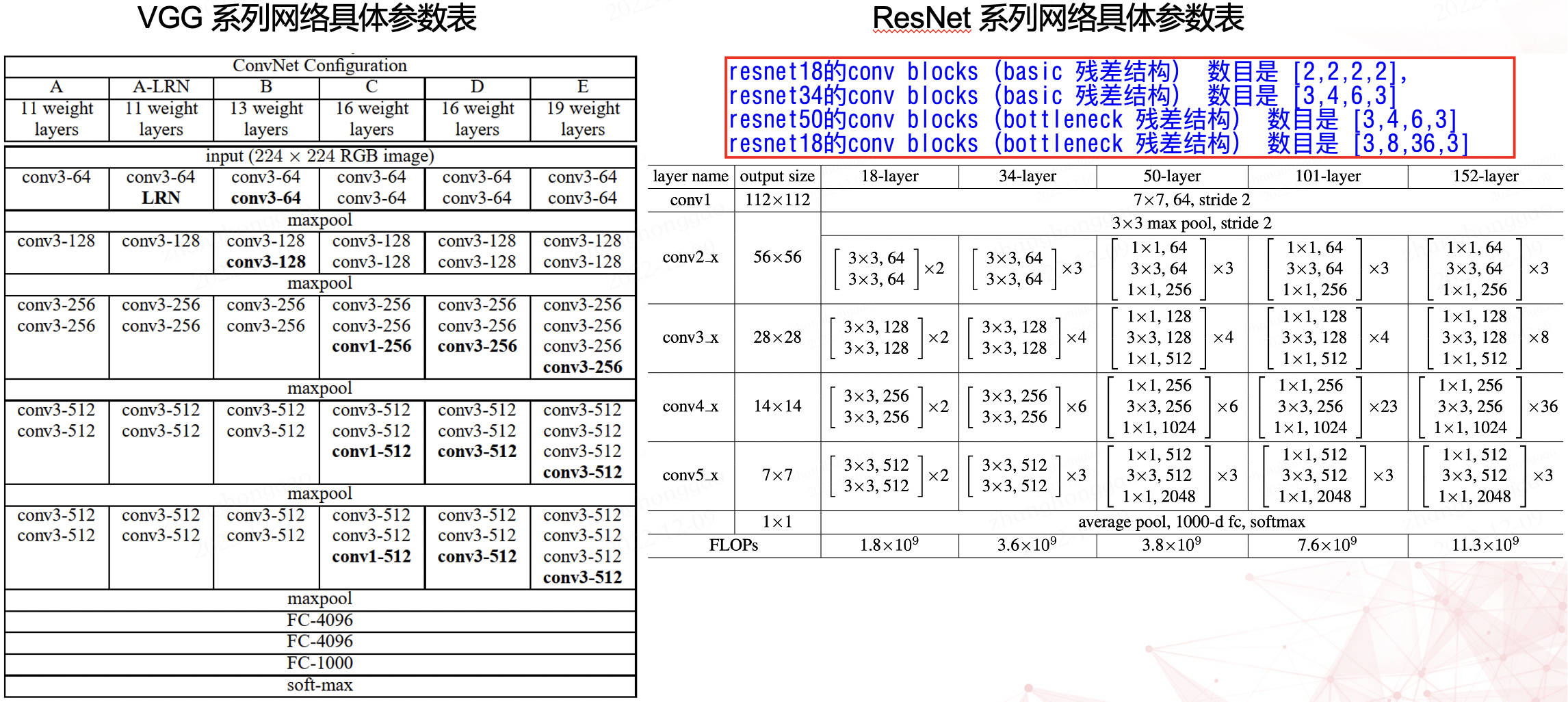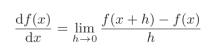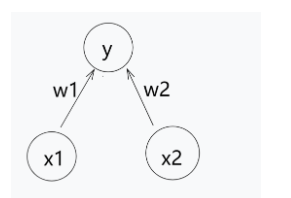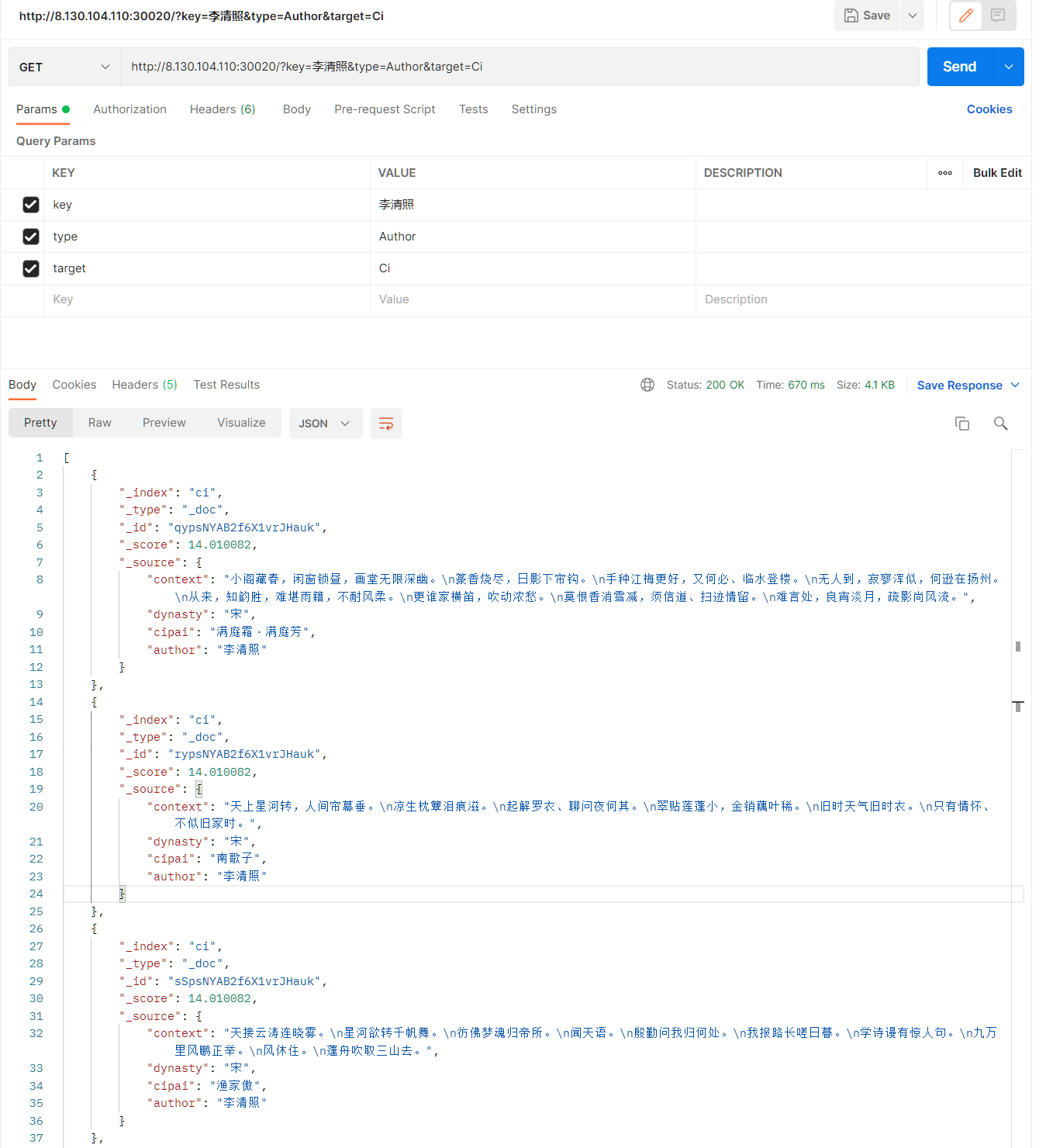# 深度学习超参数搜索实用指南

+关注继续查看## 下一个问题: 搜索代价巨大## 策略讨论

· 试错法（Babysitting

· 网格搜索（Grid Search）

· 随机搜索（Random Search）

· 贝叶斯优化（Bayesian Optimization）

## Babysitting## 网格搜索

· 定义一个N维的网格，其中每一个映射都代表一个超参数，例如，n＝（learning_rate, dropout_rate, batch_size

· 对于每个维度，定义可能的取值范围: 例如，batch_size = [4, 8, 16, 32, 64, 128, 256]

· 搜索所有可能的配置，等待结果以建立最佳配置: 例如，C1 = (0.1, 0.3, 4) ->acc = 92%, C2 = (0.1, 0.35, 4) ->acc = 92.3%···# Load the dataset
# Create model for KerasClassifier
defcreate_model(hparams1=dvalue,
hparams2=dvalue,
...
hparamsn=dvalue):
# Model definition
...
model = KerasClassifier(build_fn=create_model)
# Define the range
hparams1 = [2, 4, ...]
hparams2 = ['elu', 'relu', ...]
...
hparamsn = [1, 2, 3, 4, ...]
# Prepare the Grid
param_grid = dict(hparams1=hparams1,
hparams2=hparams2,
...
hparamsn=hparamsn)
# GridSearch in action
grid = GridSearchCV(estimator=model,
param_grid=param_grid,
n_jobs=,
cv=,
verbose=)
grid_result = grid.fit(x, y)
# Show the results
print("Best: %f using %s" % (grid_result.best_score_, grid_result.best_params_))
means = grid_result.cv_results_['mean_test_score']
stds = grid_result.cv_results_['std_test_score']
params = grid_result.cv_results_['params']
for mean, stdev, param in zip(means, stds, params):
print("%f (%f) with: %r" % (mean, stdev, param))

## 随机搜索# Load the dataset

# Create model for KerasClassifier

defcreate_model(hparams1=dvalue,

hparams2=dvalue,
...
hparamsn=dvalue):

# Model definition
...
model = KerasClassifier(build_fn=create_model)

# Specify parameters and distributions to sample from

hparams1 = randint(1, 100)

hparams2 = ['elu', 'relu', ...]
...
hparamsn = uniform(0, 1)
# Prepare the Dict for the Search
param_dist = dict(hparams1=hparams1,
hparams2=hparams2,
...
hparamsn=hparamsn)
# Search in action!
n_iter_search = 16 # Number of parameter settings that are sampled.
random_search = RandomizedSearchCV(estimator=model,
param_distributions=param_dist,
n_iter=n_iter_search,
n_jobs=,
cv=,
verbose=)
random_search.fit(X, Y)
# Show the results
print("Best: %f using %s" % (random_search.best_score_, random_search.best_params_))
means = random_search.cv_results_['mean_test_score']
stds = random_search.cv_results_['std_test_score']
params = random_search.cv_results_['params']
for mean, stdev, param in zip(means, stds, params):
print("%f (%f) with: %r" % (mean, stdev, param))## 贝叶斯优化## 高斯过程2个点的高斯过程3个点的高斯过程8个点的高斯过程

· 按照定义，这个过程是循序渐进的；

· 它只能处理数字参数；

· 如果训练表现不佳，它不提供任何机制来停止训练；

def data():
"""
Data providing function:
This function is separated from model() so that hyperopt
won't reload data for each evaluation run.
"""
# Load / Cleaning / Preprocessing
...
returnx_train, y_train, x_test, y_test
def model(x_train, y_train, x_test, y_test):
"""
Model providing function:
Create Keras model with double curly brackets dropped-in as needed.
Return value has to be a valid python dictionary with two customary keys:
- loss: Specify a numeric evaluation metric to be minimized
- status: Just use STATUS_OK and see hyperopt documentation if not feasible
The last one is optional, though recommended, namely:
- model: specify the model just created so that we can later use it again.
""
# Model definition / hyperparameters space definition / fit / eval
return {'loss': <metrics_to_minimize>, 'status': STATUS_OK, 'model': model}
# SMBO - TPE in action
best_run, best_model = optim.minimize(model=model,
data=data,
algo=tpe.suggest,
max_evals=,
trials=Trials())
# Show the results
x_train, y_train, x_test, y_test = data()
print("Evalutation of best performing model:")
print(best_model.evaluate(x_test, y_test))
print("Best performing model chosen hyper-parameters:")
print(best_run)## 早停的力量“早停”不仅是一种规则化技术，而且在训练方向不正确时，它会提供防止资源浪费的机制。· Keras提供了一个很好的“早停”功能，甚至更好的回调组件。由于Keras最近被集成在Tensorflow中，你能在Tensorflow代码中使用回调组件；

· Tensorflow提供了训练钩子，可能不像Keras回调（或tf.keras API）那么直观，但是它们提供了对执行状态的更多控制；

· 目前，Pytorch还没有提供回调组件，但是这个功能可能会随着新版本一起发布；

· fast.ai库也提供了回调功能，不过目前还没提供任何类型的文档；

· IgnitePytorch的高级库）提供类似于keras的回调，虽然还在开发阶段；

## 在FloydHub上管理实验

FloydHub的最大特性之一是，当使用不同的超参数集时能够比较正在训练的模型。FloydHub的dashboard为你提供了一种简便的方式来比较在超参数搜索中的训练任务，而且是实时更新的。我们的建议是为每一个任务创建一个不同的FloydHub项目，这样，你就可以更容易地组织工作和团队合作。

## 训练度量指标170 0114 0【深度学习】1-权重参数全相同值初始化，导致无法训练-python
【深度学习】1-权重参数全相同值初始化，导致无法训练-python
106 0159 0204 0138 0AI：人工智能概念之机器学习、深度学习中常见关键词、参数等中英文对照(绝对干货)（七）

165 0AI：人工智能概念之机器学习、深度学习中常见关键词、参数等中英文对照(绝对干货)（六）

146 0AI：人工智能概念之机器学习、深度学习中常见关键词、参数等中英文对照(绝对干货)（五）

141 0AI：人工智能概念之机器学习、深度学习中常见关键词、参数等中英文对照(绝对干货)（二）

105 0
【方向】2996121712224591

【医学搜索Query相关性判断】赛题及baseline解读249311958594

TensorRT Introduction